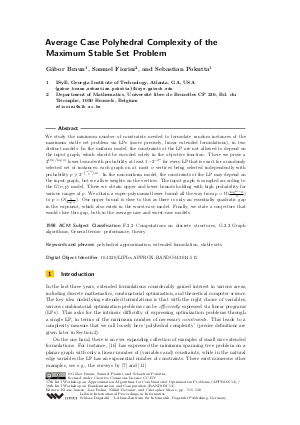Document# Average Case Polyhedral Complexity of the Maximum Stable Set Problem

### Authors Gábor Braun, Samuel Fiorini, Sebastian Pokutta## File

LIPIcs.APPROX-RANDOM.2014.515.pdf
• Filesize: 0.54 MB
• 16 pages

## Cite As

Gábor Braun, Samuel Fiorini, and Sebastian Pokutta. Average Case Polyhedral Complexity of the Maximum Stable Set Problem. In Approximation, Randomization, and Combinatorial Optimization. Algorithms and Techniques (APPROX/RANDOM 2014). Leibniz International Proceedings in Informatics (LIPIcs), Volume 28, pp. 515-530, Schloss Dagstuhl - Leibniz-Zentrum für Informatik (2014)
https://doi.org/10.4230/LIPIcs.APPROX-RANDOM.2014.515

## Abstract

We study the minimum number of constraints needed to formulate random instances of the maximum stable set problem via LPs (more precisely, linear extended formulations), in two distinct models. In the uniform model, the constraints of the LP are not allowed to depend on the input graph, which should be encoded solely in the objective function. There we prove a super-polynomial lower bound with overwhelming probability for every LP that is exact for a randomly selected set of instances with a natural distribution. In the non-uniform model, the constraints of the LP may depend on the input graph, but we allow weights on the vertices. The input graph is sampled according to the Erdös-Renyi model. There we obtain upper and lower bounds holding with high probability for various ranges of p. We obtain a super-polynomial lower bound all the way from essentially p = polylog(n) / n to p = 1 / log n. Our upper bound is close as there is only an essentially quadratic gap in the exponent, which also exists in the worst case model. Finally, we state a conjecture to close the gap both in the average-case and worst-case models.
##### Keywords
• polyhedral approximation
• extended formulation
• stable sets

## Metrics

• Access Statistics
• Total Accesses (updated on a weekly basis)
0